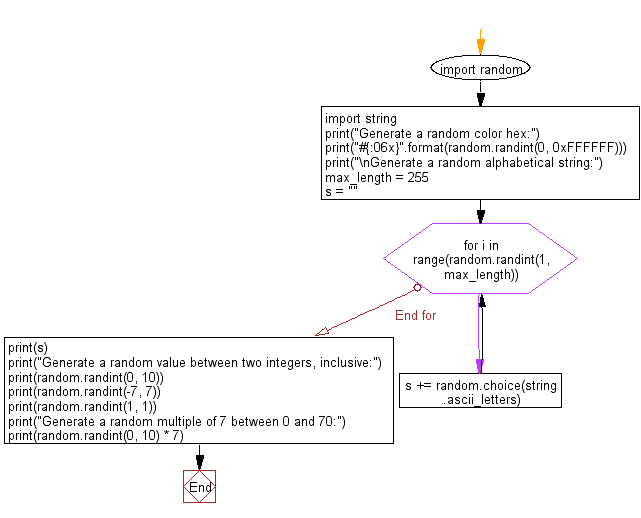﻿ Python: Generate a random color hex, alphabetical string, random value and multiple of 7 - w3resource# Python: Generate a random color hex, alphabetical string, random value and multiple of 7

## Python module: Exercise-1 with Solution

Write a Python program to generate a random color hex, a random alphabetical string, random value between two integers (inclusive) and a random multiple of 7 between 0 and 70.

Use random.randint()

Sample Solution:

Python Code:

``````import random
import string
print("Generate a random color hex:")
print("#{:06x}".format(random.randint(0, 0xFFFFFF)))
print("\nGenerate a random alphabetical string:")
max_length = 255
s = ""
for i in range(random.randint(1, max_length)):
s += random.choice(string.ascii_letters)
print(s)
print("Generate a random value between two integers, inclusive:")
print(random.randint(0, 10))
print(random.randint(-7, 7))
print(random.randint(1, 1))
print("Generate a random multiple of 7 between 0 and 70:")
print(random.randint(0, 10) * 7)
```
```

Sample Output:

```Generate a random color hex:
#eb76d4

Generate a random alphabetical string:
lGhPpBDqfCgXKzSbGcnmcDWBEZeiqcUqztgvwcXfVyPslOggKdbIxOejJfFMgspqrgskanNYpscJEOVIpYkGGNxQlaqeeubGDbQSBhBedrdOyqOmKPTZvzKmKVoidsuShSCapEXxxNJRCxXOwYUUPBefKmJiidGxHwvOxAEujZGjJjTqjRtuEXgyRsPQpqlqOJJjKHAPHmIJLpMvLTRVqwSeLCIDRdMnYpbg
Generate a random value between two integers, inclusive:
0
4
1
Generate a random multiple of 7 between 0 and 70:
70
```

Flowchart:## Visualize Python code execution:

The following tool visualize what the computer is doing step-by-step as it executes the said program:

Python Code Editor:

Have another way to solve this solution? Contribute your code (and comments) through Disqus.

What is the difficulty level of this exercise?

Test your Python skills with w3resource's quiz

﻿

## Python: Tips of the Day

Dictionary comprehension:

```>>> m = {x: x ** 2 for x in range(5)}
>>> m
{0: 0, 1: 1, 2: 4, 3: 9, 4: 16}
```